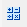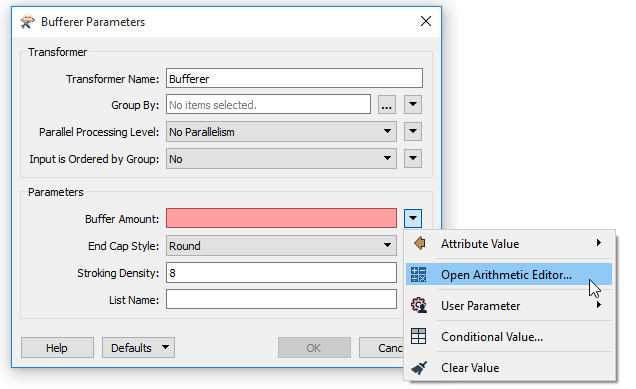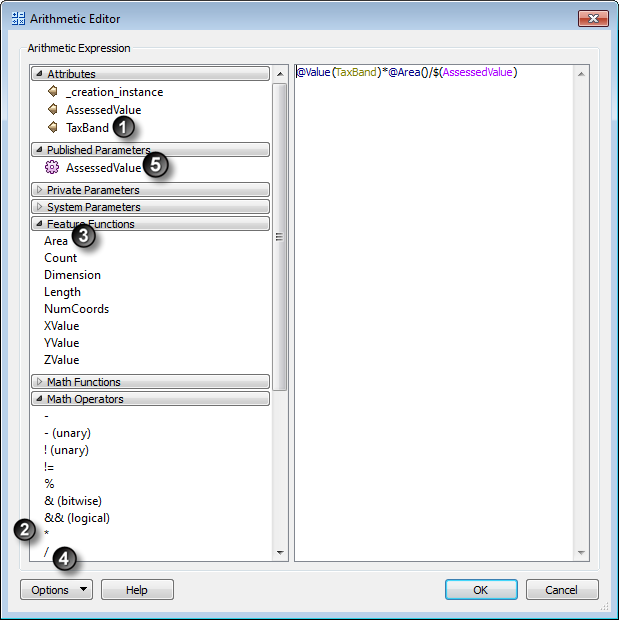You are here: Transforming Data > Working with Transformer Parameters > Arithmetic Editor

# Arithmetic EditorThe Arithmetic Editor provides a convenient way to construct math expressions from various data sources, such as attributes, parameters, and feature functions, where the result is used directly inside a parameter.

For example, you might wish to calculate the size of a buffer from various other attributes, functions, or parameters.

In this example, the source data has a vehicle speed attribute for each point. The data is converted into point features, and the individual buffers are then merged into a single area feature. You want to show areas where vehicle speed is low, to indicate traffic congestion zones.

By using the Arithmetic Editor, you can directly attach the calculation to the Buffer Amount parameter:Using the Arithmetic Editor, you can create a buffer around each point, where buffer radius = speed * 4:Similarly, in the AttributeCreator transformer, you could create an attribute such as a property’s Taxable Value using an expression such as `(@Value(TaxBand) * @Area())/\$(AssessedValue)`.

The numbers 1-5 show the order in which the elements were added to the editor:## Attributes

After the transformer is connected in the workspace, this section lists the available attributes. You can use attributes to supply a value for use within the arithmetic expression.

## Published/Private Parameters

This is a list of the published/private parameters (if any) that are in the workspace. You can use published parameters to supply a user-defined value for use within the arithmetic expression.

## FME Parameters

In some scenarios, FME Parameters may be useful in the workspace.

## FME Feature Functions

These functions are capable of returning a numeric value, which is substituted into the arithmetic expression where defined.